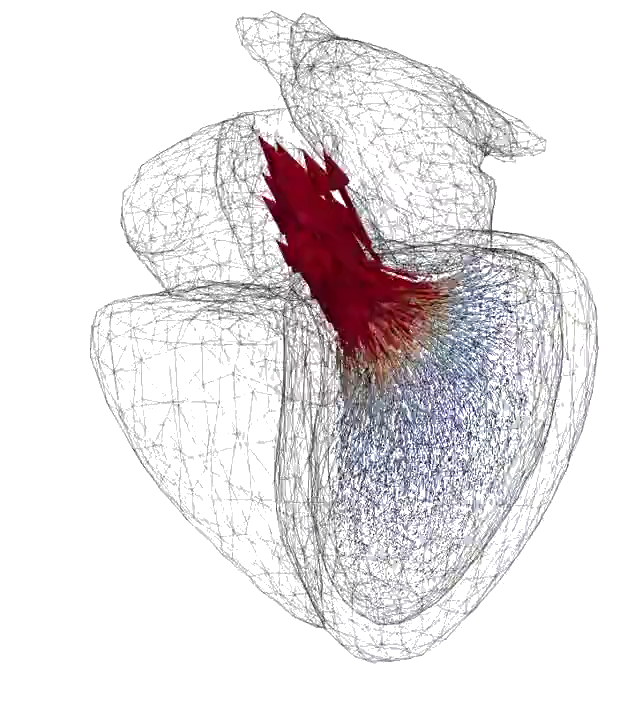#### — Learn Mathematical Programming!#### — Learn Mathematical Programming!

• New revolutionary way to learn math + coding!
• For all levels.
• High impact activities developed by leading scientists.

Contact: Johan Jansson jjan@kth.se

DigiMat is a spin-off of the Digital Math research environment: http://digitalmath.tech .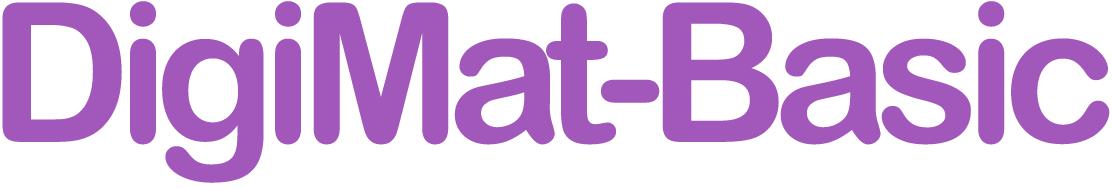DigiMat Basic is for you who have no or little experience in Mathematical Programming. Mathematician Ada Lovelace (1815-1852) will guide you through her wonderful world! DigiMat Basic consists of:

• Ada's World — Learn the binary addition algorithm by throwing a party!

• Ada's Friends — Meet and be inspired by Ada's friends, important scientists from history, and explore how mathematical programming can be used in different contexts.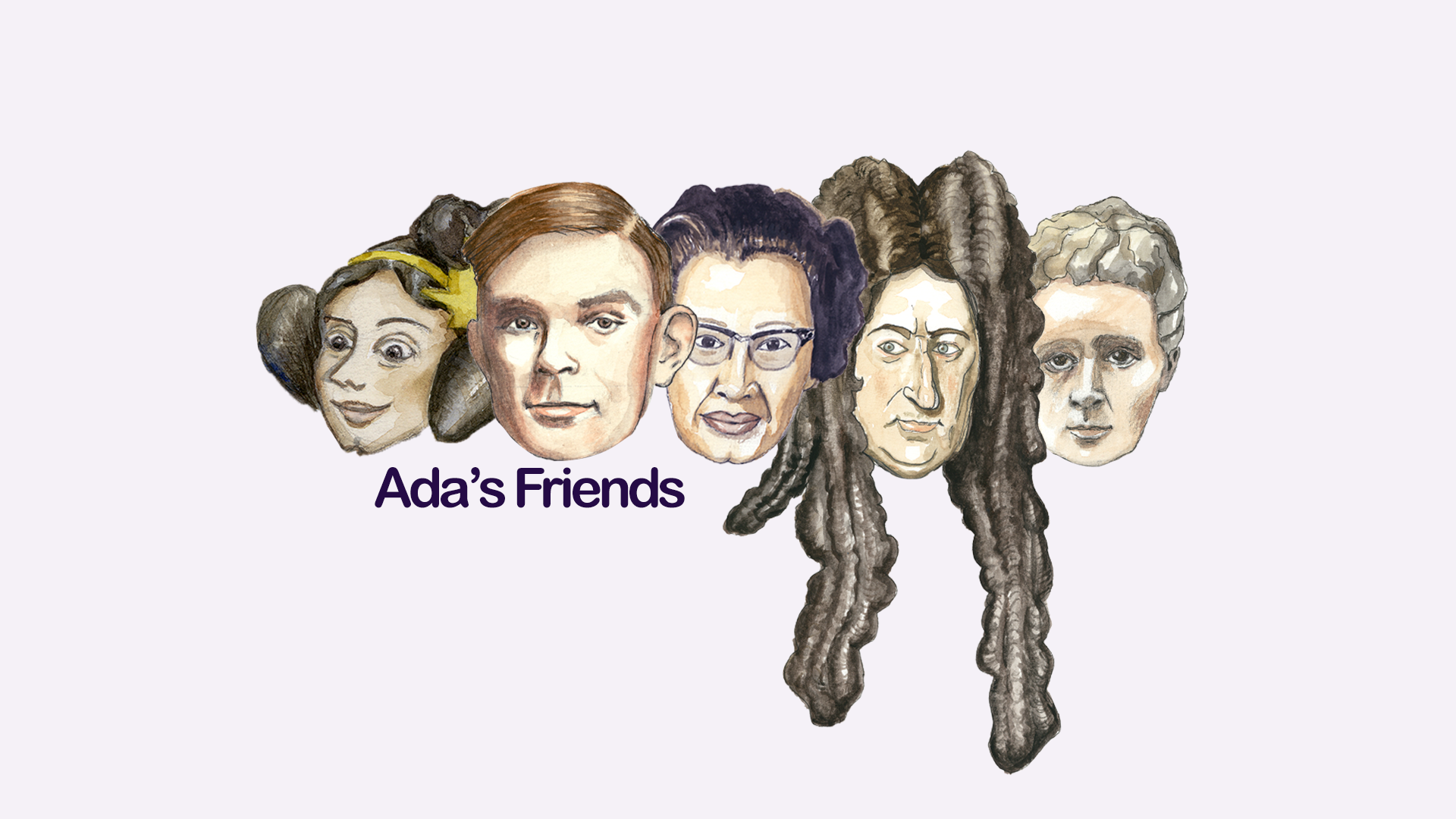• DigiMat Pixel — Create digital objects and give them life!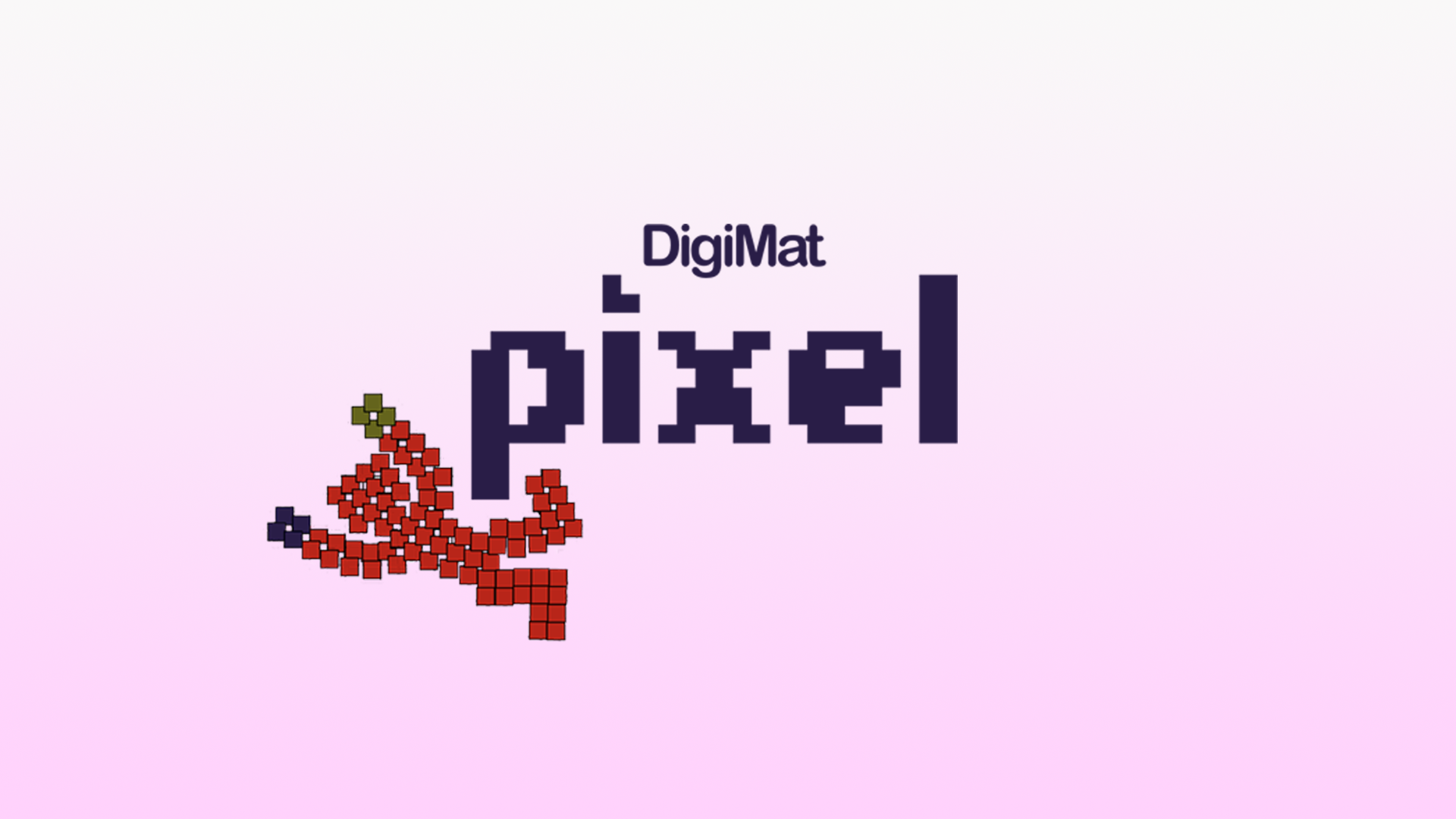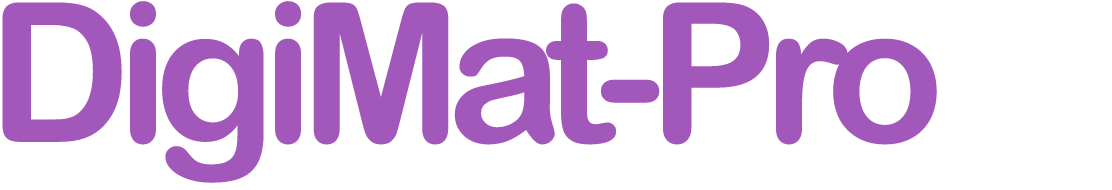• This is the advanced DigiMat course for university level, professionals and experienced programmers.
• Get started here and now! You'll find the entire course with videos and interactive learning activities in the modules below.
• To receive a course certificate for a fee of 100 USD, e-mail course leader Johan Jansson, jjan@kth.se. Or pay directly through Stripe with this link: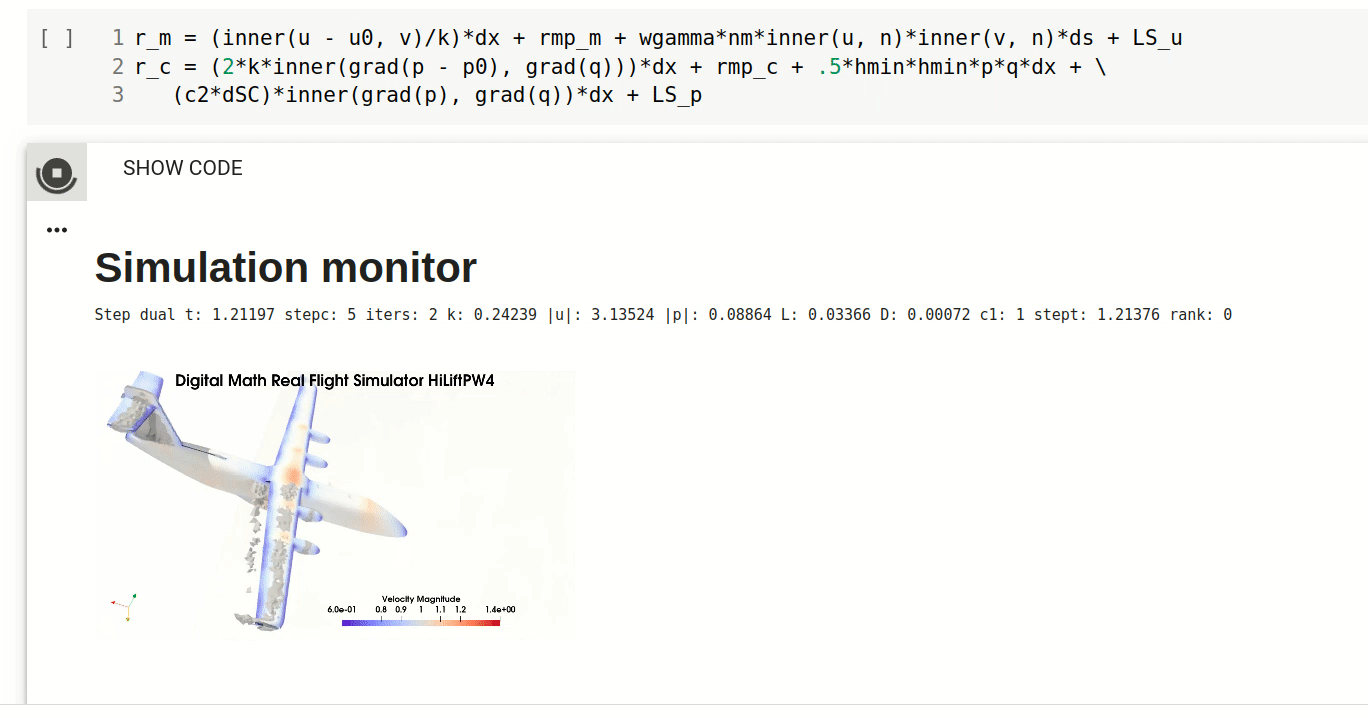Example of DigiMat Pro environment

1. Understand and try how Digital Math underlies science and industry, and allows prediction of the mathematical models governing reality.

2. Galerkin's method part 1. Understand the finite element method in a familiar linear algebra setting. Learn how the finite elelement method is the best possible method for several classes of problems.

3. Adaptive error control. Learn how to optimally apply the finite element discretization with adaptivity for fast and cheap computation.

4. Get an overview of high performance and advanced predictive aerodynamics applications and learn about performance in an adaptive FEM and HPC setting.

5. Understand the mechanism of flight, aerodynamics and turbulence and how to efficiently predict flight simulation with Real Flight Simulation in Digital Math.

#### You can also enter DigiMat Pro in the CMU-OLI system in collaboration with Carnegie-Mellon Open Learning Initiative.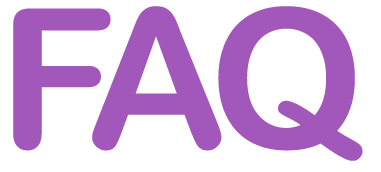## What is Mathematical Programming?

Mathematical programming unifies math and coding. It’s based on scientifically grounded physics simulations, which means computing and predicting scenarios with a computer. With DigiMat you’ll learn to create your own virtual worlds.

## Who is DigiMat for?

DigiMat is for everyone, no matter age or level. Kids, parents, teachers and university students and professionals. Anyone can learn to use the simple algorithms of DigiMat.

## Why learn Mathematical Programming?

Mathematical Programming is crucial for industry and our digital society. With mathematical programming you can do much more powerful, interesting and useful things than with regular coding.

## What didactic method does DigiMat use?

DigiMat focuses on motivation, creativity and learning by doing. Motivation is key to store information in the long term memory. By focusing on the amazing things you can achieve you'll find motivation to learn more and reach higher.

With mathematical programming you get a better understanding of the algorithms rather than learning special cases by heart, which is what traditional math education often focus on. You learn that the basics of math and coding are the same, and by that you understand the basic principles of both mathematics and programming.

## Which are the DigiMat learning goals?

1. Number representation [Basic] in first binary form making representation and arithmetic algorithms easy to understand.
2. Arithmetic algorithms [Basic] constructed by repetition of the basic operation of +1 according the basic prototype of all computer programs of DigiMat in the form n = n + 1
3. Time-stepping [Basic-Pro] automatically solving all (ordinary) mathematical models in the form x = x + v*dt
4. Text programing [Basic-Pro] enabling the students to understand, modify and extend the algorithms and computer realizations themselves.